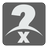Number Guess
Elementary+
English

...When you have eliminated all which is impossible, then whatever remains, however improbable, must be the truth.
Sir Arthur Conan Doyle - Sherlock Holmes

You are given an unknown number within the range of 1 to 100, inclusive. Your task is to guess what the number is by performing a series of guesses .

Your solution will need to guess the number by submitting an integer divisor ranging from 2 to 10, and the number you guessed . At each attempt you will get information as a list of tuples. Each tuple contains the remainder result along with your previous divisor.

You should find the number within 8 guesses. We will give you a little extra info on your first attempt... Use it wisely young grasshopper ...

Consider the following example:

 Attempt Divisor Guess Submit Information Remark 1 2 99 (2,99) (1,5),(1,2) You try a divisor of 2 and try your luck with 99; however, you've got... 3 6 41 (6,41) (1,5),(1,2),(2,3),(5,6) Your solution might suggest 41 because it passed with the information data. ( 41%5=1; 41%2=1; 41%3=2 ) Again, you've still got no response. 4 4 71 (4,71) (1,5),(1,2),(2,3),(5,6),(3,4) Your solution may suggest 71 because it passed with the information data. ( 71%5=1; 71%2=1; 71%3=2; 71%6=5 ) Now there are only 4 remaining attempts left. 5 and, so on... 6 7 8
 Attempt Divisor Guess Submit Response 1 2 99 (2,99) (1,5),(1,2) Remark #1: You try a divisor of 2 and try your luck with 99; however, you've got a response of a list of tuples, returning the remainder of your previous divisor and your previous divisor. ( that means you still need to guess ) 2 3 91 (3,91) (1,5),(1,2),(2,3) Remark #2: Your solution suggests 91 because it passed with the response data, isn't it? ( 91%5=1; 91%2=1 ) Again, you've still got a response. Next guess, then. 3 6 41 (6,41) (1,5),(1,2),(2,3),(5,6) Remark #3: Your solution suggests 41 because it passed with the response data. ( 41%5=1; 41%2=1; 41%3=2 ) Again, you've still got a response. 4 4 71 (4,71) (1,5),(1,2),(2,3),(5,6),(3,4) Remark #4: Your solution suggests 71 because it passed with the response data. ( 71%5=1; 71%2=1; 71%3=2; 71%6=5 ) Again, you've still got a response. 5 and, so on... 6 7 8

You get the idea...

Input: Information about the previous attempt. A list of tuples. Each tuple contains its remainder and the previous divisor.

Output: A list of integer divisors and your best guess. A list of two integers.

Example:

```checkio([(1,5)])                            # the number has a remainder 1
checkio([(1,5),(1,2)])                      # the number has a remainder 1
checkio([(1,5),(1,2),(2,3)])                # the number has a remainder 2
checkio([(1,5),(1,2),(2,3),(5,6)])          # the number has a remainder 5
checkio([(1,5),(1,2),(2,3),(5,6),(3,4)])    # the number has a remainder 3
```

How it is used: This is a classical prediction and decision problem. You have some indirect information and should to get hidden by it data. The skills that go into this problem could help you create a sports bracket for the office pool.

Precondition:
0 < number ≤ 100

You should be an authorized user in order to see the full description and start solving this mission.